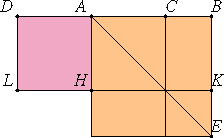# Proposition 5

If a straight line is cut in extreme and mean ratio, and a straight line equal to the greater segment is added to it, then the whole straight line has been cut in extreme and mean ratio, and the original straight line is the greater segment.

Let the straight line AB be cut in extreme and mean ratio at the point C, let AC be the greater segment, and let AD be equal to AC.I say that the straight line DB is cut in extreme and mean ratio at A, and the original straight line AB is the greater segment.

I.46

Describe the square AE on AB, and draw the figure.

Since AB is cut in extreme and mean ratio at C, therefore the rectangle AB by BC equals the square on AC.

And CE is the rectangle AB by BC, and CH is the square on AC, therefore CE equals HC.

But HE equals CE, and DH equals HC, therefore DH also equals HE. Therefore the whole DK is equal to the whole AE.

And DK is the rectangle BD by DA, for AD equals DL, and AE is the square on AB, therefore the rectangle BD by DA equals the square on AB.

Therefore DB is to BA as BA is to AD. And DB is greater than BA, therefore BA is also greater than AD.

Therefore DB has been cut in extreme and mean ratio at A, and AB is the greater segment.

Therefore, if a straight line is cut in extreme and mean ratio, and a straight line equal to the greater segment is added to it, then the whole straight line has been cut in extreme and mean ratio, and the original straight line is the greater segment.

Q.E.D.

## Guide

#### Use of this proposition

This proposition is used XIII.17 where a dodecahedron is constructed.